Bells, Braids, and Taxicabs

loopspace

# 1 Introduction

This is a short journey through some regions of Mathematics inspired by a seemingly-random question from Catriona Shearer on twitter that flew past me on twitter one day. It starts with a morning of bell ringing, spends some time braiding, and ends the journey with a mad dash in a taxi.

# 2 Bell Ringing

Those around me have gotten used to me declaring "But that's Mathematics" to the point that they are quick to reply "Yes, but you say that everything is Mathematics.". Which is fair enough. I do have a tendency to think that. But with bell ringing, I'm not on my own in this claim.

I should confess at the outset that I know nothing about bell ringing itself. All I know are its connections to Mathematics. So I'll shift to the Mathematics straightaway to avoid demonstrating my lamentable ignorance.

The bells are rung in rounds which consist of ringing each bell in a given order. In successive rounds the order can be changed but only to the extent of two neighbouring bells swapping position in the order (more than two pairs can swap between each round). (It is possible for the last bell of one round to swap with the first bell of the next but this isn't usually done.)

As an example, here's a few rounds with five bells.

 $\begin{array}{ccccc}1& 2& 3& 4& 5\\ 2& 1& 4& 3& 5\\ 2& 4& 1& 5& 3\\ 4& 2& 5& 1& 3\end{array}$

"Ringing the Changes" refers to doing a succession of swaps (with just one pair swapping each time) which traverses the full set of permutations. That isn't what I'm interested in.

# 3 Braids

When listing a sequence of rounds, it can be helpful to illustrate the changes by drawing lines following each bell. Figure 1 shows what the above rounds would look like.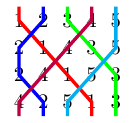Figure 1: Joining the bells

A few sources that I've read refer to this as a braid. It isn't. Despite the temptation of both braids and bells being about ropes, simply tracing the location of each bell does not make it a braid. To be a braid, we need to include information as to which strand crosses over which at each crossing. In bell ringing, this has no interpretation since two bells simply swap position in the round: they don't actually swap (as amusing it might be to make the ringers swap position, the tangling of the ropes would quickly make it so that the bells didn't chime).

That shouldn't stop us playing with the idea, though. We just need to determine a rule for setting the crossings. Some to play around with are:

1. For each pair of numbers, pick a "winner" and make that one always cross over the other.

For example, with five strands we could simulate Rock, Paper, Scissors, Lizard, Spock.

2. Similar to the above, but rather than deciding by strand, decide by current position.

3. Make all crossings left over right.

4. Make a choice for one crossing, then choose the others so that the braid is alternating.

There are then numerous questions that one could ask about these braids. I'll pose just one before getting on with this story.

Question

For this to work, the final order of the bells needs to be the same as its initial order.

Using the first rule for deciding the crossings, since for each pair one particular strand always passes over the other, then any pair of strands are unlinked. To what extent does this hold for more sets of strands?

If it is the case that removing any one strand leaves all the others unlinked then the braid is known as Brunnian.

# 4 Taxis

The motivating question for this journey relates to the area swept out between two strands as the braid progresses. (It is worth pointing out that this doesn't depend on the choices of crossings in the braid.) The original problem also imposed a "must swap if possible" rule but we don't have to assume that.

Let's take a look at a diagram created with five strands which progresses by repeated application of two moves. The first move swaps the strands in positions $1$ and $2$ and in $3$ and $4$. The second swaps the strands in positions $2$ and $3$ and in $4$ and $5$. A full sequence takes ten moves to return to the beginning and is shown in Figure 2 (with the over–unders chosen so that the crossings make the braid alternating).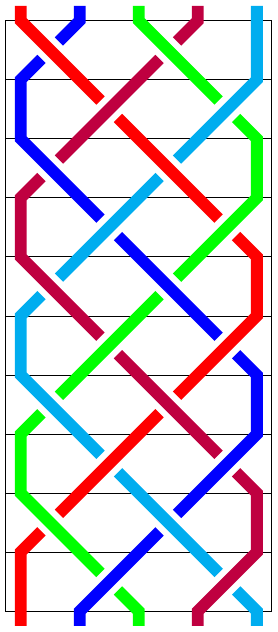Figure 2: A five strand swap

Let's look at a part of this. At the first change, let's look at the blue and green strands (bells $2$ and $3$). To talk about area, we need to decide on units, so let's assign length $1$ to every atomic length (i.e., the horizontal distance between strands and the vertical distance between the lines that correspond to the bells being played). So the part of first change concerning the blue and green strands looks like Figure 3.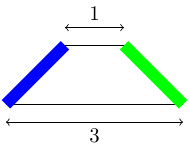Figure 3: A single piece

This is a trapezium, so its area is straightforward to calculate. Our choice of units means that the area is the average of the two horizontal distances. More generally, the area of most sections will be the average of their horizontal distances. The only exceptions are where there are crossings, as in Figure 4.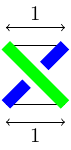Figure 4: A crossing

When there is a crossing, the area given by the average of their horizontal distances is $1$ but the area between the lines is half that. Alternatively, we can write it as $1-\frac{1}{2}$, where the $1$ corresponds to the area given by the "average of their horizontal distances" rationale and the $\frac{1}{2}$ is the correction from the crossing.

To put this all together we need to establish some notation. It is traditional to use greek lowercase letters such as $\sigma$ to denote permutations, so let us write ${\sigma }_{i}$ for the permutation of the bells at the $i$th level, with ${\sigma }_{0}$ as the identity. Standard notation is to write, for example, $1{\sigma }_{3}$ for the position of the first strand at the third level, so in Figure 2 then $1{\sigma }_{3}=4$.

The distance between two strands is therefore the sum of terms like:

 $\frac{1}{2}|i{\sigma }_{k-1}-j{\sigma }_{k-1}|+\frac{1}{2}|i{\sigma }_{k}-j{\sigma }_{k}|$

with a correction factor of $\frac{1}{2}$ for each crossing. In the sum, $k$ varies but $i$ and $j$ are held fixed. What is important to note is that the second part of the term for $k$ is equal to the first part of the next term for $k+1$. That means that with the exception of the start and end, all the $\frac{1}{2}$s will add up to $1$s. So the total area is:

 $\frac{1}{2}|i{\sigma }_{0}-j{\sigma }_{0}|+{\sum }_{k=1}^{n-1}|i{\sigma }_{k}-j{\sigma }_{k}|+\frac{1}{2}|i{\sigma }_{n}-j{\sigma }_{n}|-{\chi }_{i,j}$

where ${\chi }_{i,j}$ is half the number of crossings between strands $i$ and $j$.

Let us make the assumption that our bell ringers end up where they started. This means that ${\sigma }_{n}={\sigma }_{0}$ and our formula therefore simplifies to:

 ${\sum }_{k=0}^{n-1}|i{\sigma }_{k}-j{\sigma }_{k}|-{\chi }_{i,j}$

Note that if we use a crossing rule that ensures that the crossings between any two strands alternate, then ${\chi }_{i,j}$ is the linking number of those two strands. One way to achieve this property is to make the crossings all the same way, for example, left over right. Another, for the braid of Figure 2, is shown in Figure 5. Regardless, ${\chi }_{i,j}$ is the maximum linking number over all possible ways of deciding the crossings.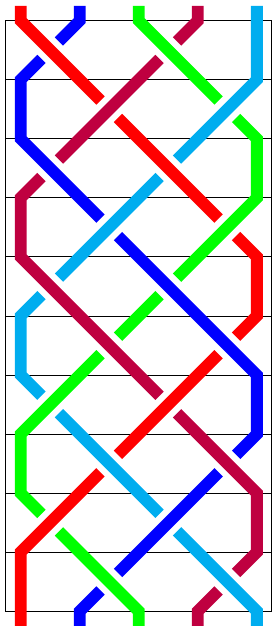Figure 5: A five strand swap

So the second term is a measure of how much the two strands cross each other.

The first term is where the taxis arrive.

The taxicab metric, more formally known as the ${\ell }^{1}$–metric, is a way of measuring distance between points. In $2D$, the image is of points laid out on a grid, much like a city such as New York, as in Figure 6. You can move along the lines on the grid but you can't move across them. The distance between two points is therefore not the standard Euclidean distance but a different formula. More prosaically, it is not "as the crow flies" but rather "as the taxi drives".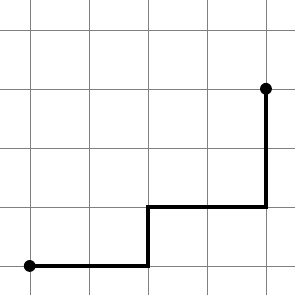Figure 6: Grid distances

The distance between the two points in Figure 6 is $2+1+2+2=7$. No matter what choice of route, no shorter distance can be found. This gives rise to the formula taxicab distance between two points as:

 $\parallel a-b{\parallel }_{1}=|{a}_{x}-{b}_{x}|+|{a}_{y}-{b}_{y}|$

There's nothing special about $2D$ here. The analogous formula holds with arbitrary length vectors. Thinking of a vector as just a list of numbers (i.e., without trying to assign any physical meaning to the directions), we can get just such a vector from a strand of our braid by listing the positions. Thus in our running example, the red strand gives rise to the vector:

 $\left(1,2,3,4,5,5,4,3,2,1\right)$

Let us write ${v}_{i}$ for the vector arising from the strand that starts in position $i$. Then the area formula simplifies to

 $\parallel {v}_{i}-{v}_{j}{\parallel }_{1}-{\chi }_{i,j}$

If we list all the vectors arising from Figure 2, we see some more structure:

 $\begin{array}{rl}{v}_{1}& =\left(1,2,3,4,5,5,4,3,2,1\right)\\ {v}_{2}& =\left(2,1,1,2,3,4,5,5,4,3\right)\\ {v}_{3}& =\left(3,4,5,5,4,3,2,1,1,2\right)\\ {v}_{4}& =\left(4,3,2,1,1,2,3,4,5,5\right)\\ {v}_{5}& =\left(5,5,4,3,2,1,1,2,3,4\right)\end{array}$

Each vector is a cyclic permutation of the others. That is, if we define the operation $\tau$ on vectors to be the operation which cycles the components around, we have:

 $\begin{array}{rl}{v}_{2}& ={\tau }^{2}{v}_{1}\\ {v}_{3}& ={\tau }^{-2}{v}_{1}\\ {v}_{4}& ={\tau }^{4}{v}_{1}\\ {v}_{5}& ={\tau }^{-4}{v}_{1}\end{array}$

This reduces the number of calculations we have to do as we can use the fact that the taxicab metric gives the same answer if both vectors are rotated by the same amount. We therefore only have to calculate:

 $\begin{array}{rl}\parallel {v}_{1}-{v}_{2}{\parallel }_{1}& =16\\ \parallel {v}_{1}-{v}_{4}{\parallel }_{1}& =24\end{array}$

From this, we can work out all the areas swept out between different strands (noting that the contribution from crossings is $-1$ in each case). We get:

 $\begin{array}{rl}A\left({v}_{1},{v}_{2}\right)=A\left({v}_{2},{v}_{4}\right)=A\left({v}_{4},{v}_{5}\right)=A\left({v}_{5},{v}_{3}\right)=A\left({v}_{3},{v}_{1}\right)& =15\\ A\left({v}_{1},{v}_{4}\right)=A\left({v}_{4},{v}_{3}\right)=A\left({v}_{3},{v}_{2}\right)=A\left({v}_{2},{v}_{5}\right)=A\left({v}_{5},{v}_{1}\right)& =23\end{array}$

In conclusion, the area between two strands is given by the difference of the taxicab metric applied to the corresponding vectors, and the maximum linking number between the strands.

# 5 Conclusion

As with the majority of my mathematical journeys these days, this started with an intriguing problem, then began travelling through areas that I thought I knew before emerging in another completely different region which I never believed would be connected to the others. This particular journey hasn't taught me any new mathematical knowledge, but by linking together topics that I hadn't thought of together, it's given me a whole new set of things to ponder. I'm now curious as to what patterns can emerge from these ringing patterns. More investigation is clearly needed!

Plus it gives me an excuse to draw some more braid diagrams, which is always a bonus.